# Python Matplotlib drawing violin diagram

2022-02-01 12:07:55This is my participation 11 The fourth of the yuegengwen challenge 25 God , Check out the activity details ：2021 One last more challenge

# Review

matplotlib yes Python A library dedicated to rendering , We also learned before pyplot Provides drawing polylines 、 Scatter 、 Columnar 、 Square 、 Pie charts and other common images , Also provide animation Class to draw dynamic graph . At the same time, for special charts, such as quantum field diagrams applied in Physics 、 Pay attention to the box diagram of data distribution, etc . Examples of previous articles mentioned above are as follows .

Following the last time we learned that we can quickly display the median of a set of data 、 The upper and lower quartiles 、 Box diagram of maximum and minimum value pyplot.boxplot() Method . There is also a graphical violin diagram showing the data distribution .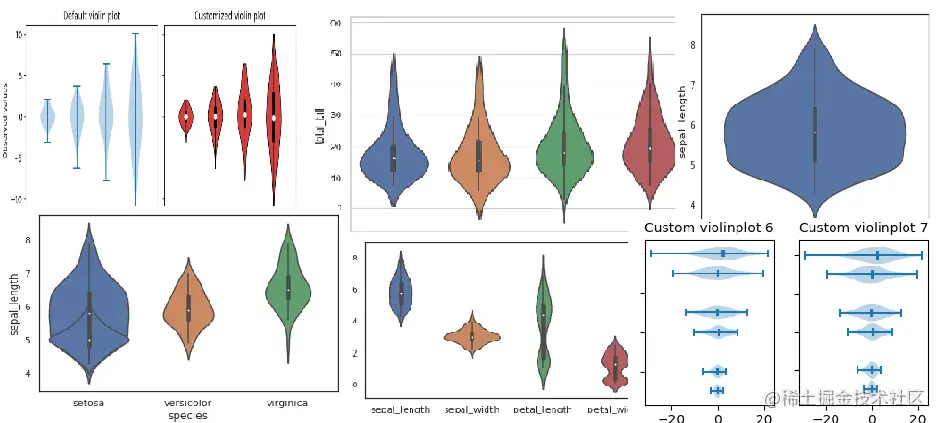In this issue , We learn matplotlib.pyplot.violinplot Draw violin chart and learn related attributes ,Let's go~

# 1. Violin overview

• ## What is the violin picture ？

• The picture of violin is similar to the shape of violin , It has the characteristics of box diagram and density diagram
• Violin chart is used to show the density of data distribution and occurrence probability
• The violin chart shows the median of the same set of data 、 Quartile range 、 density 、95% confidence interval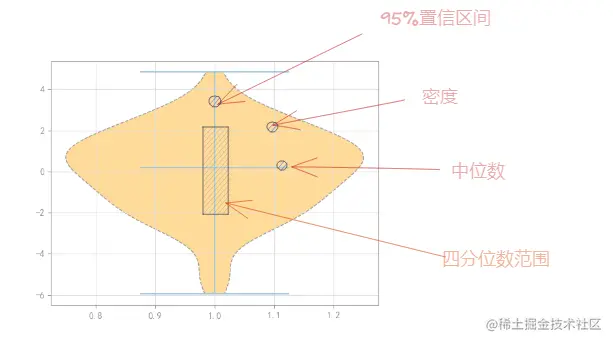• ## Violin diagram application scenario

• The violin diagram can be used to compare the density distribution between different samples
• The violin chart can be used to show the complete distribution of data
• Violin charts are often used to sort out discrete data before making accurate charts
• Cello charts are usually used for gene sample distribution 、 Population age distribution, etc
• ## How to get violin diagram

``````import matplotlib.pyplot as plt
plt.violinplot(dataset)
Copy code ``````

# 2. Violin chart properties

• ## Set the violin map position

• keyword ：vert
• The default value is ：True
• When vert by True Create a vertical violin diagram
• When vert by False Create a horizontal violin diagram
• ## Set the violin width

• keyword ：width
• The default value is ：0.5
• ## Set violin data

• Set the keyword to display the mean value ：showmeans, The default is False
• Set the keyword to display extreme value ：showextrema, The default is True
• Set the keyword to display the median ：showmedians, The default is False
• ## Set violin style

• Need to use pyplot.violinplot() Return field “bodies”
• combination for Loop traversal pyplot.violinplot()["bodies"]
• call set_facecolor Set the violin color
• call set_edgecolor Set the violin border color
• call set_alpha Set the transparency of the violin chart
• call set_linestyle Set the violin border style

# 3. To draw a violin diagram

• Import matplotlib.pyplot class
``````import matplotlib.pyplot as plt
Copy code ``````
• Use numpy In the database normal(),random(),randint() And so on data Array vector sequence
``````data = [np.random.normal(0,2,100)]
Copy code ``````
• call pyplot.violinplot(data) Method to draw the violin
``````part = plt.violinplot(data,showmedians=True)
Copy code ``````
• call pyplot.show() Method to render and display the violin chart
``````plt.show()
Copy code ``````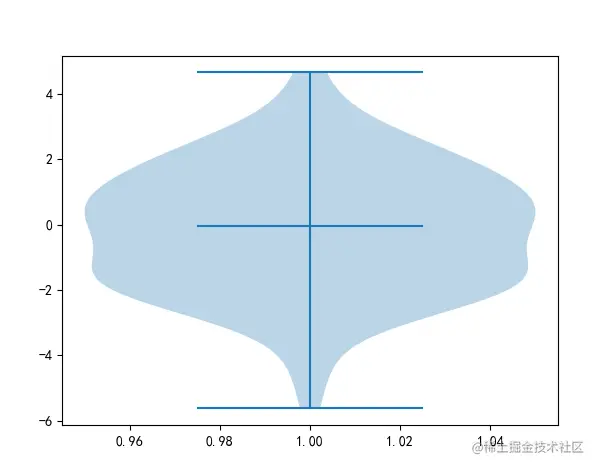• By using yplot.violinplot() Method return value , Set the color of the violin chart 、 Border styles, etc
``````for pc in part["bodies"]:
print(pc)
pc.set_facecolor("pink")
pc.set_edgecolor("black")
pc.set_alpha(1)
pc.set_linestyle("--")
Copy code ``````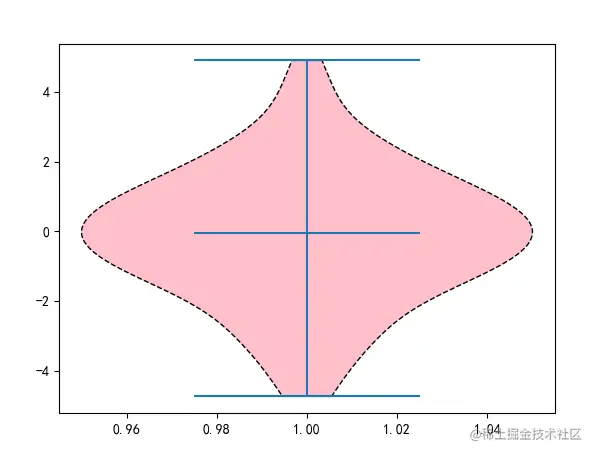# 4. A profound

We learn to matplotlib The drawing of violin provided violinplot() After the method , Let's practice with scatter combination

• call pyplot.grid() Method to add a mesh to the canvas
• call numpy.percentile() Calculate the next quarter into numbers , Mean number , The upper quarter is divided into numbers
• call pyplot.scatter() Mark the sign of the mean on the violin chart
• call pyplot.vline() Mark the quartile on the violin chart
``````data = [np.random.normal(0,i,100) for i in range(2,6)]
plt.grid()
part = plt.violinplot(data,showmedians=True,widths=[0.3,0.4,0.5,0.6])
for pc in part["bodies"]:
print(pc)
pc.set_facecolor("orange")
pc.set_edgecolor("black")
pc.set_alpha(1)
pc.set_linestyle("--")

q1,me,q3 = np.percentile(data,[25,50,75],axis=1)
ind = np.arange(1,len(me)+1)

plt.scatter(ind,me,marker="o",color="r",s=50,zorder=3)
plt.vlines(ind,q1,q3,color="k",linestyles="-",lw=5)
Copy code ``````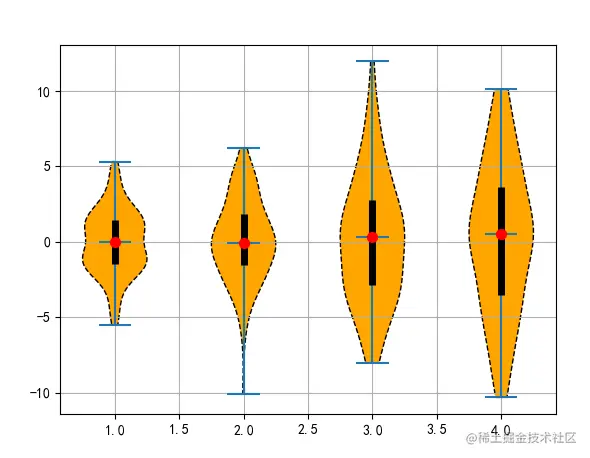# summary

In this issue , We are right. matplotlib.pyplot.violinplot() Method to draw violin diagram related attributes . Violin diagram combines the characteristics of box diagram and density diagram , Not only show the distribution of data , It also shows the density of the data .

The above is the content of this issue , Welcome big guys to praise and comment , See you next time ~